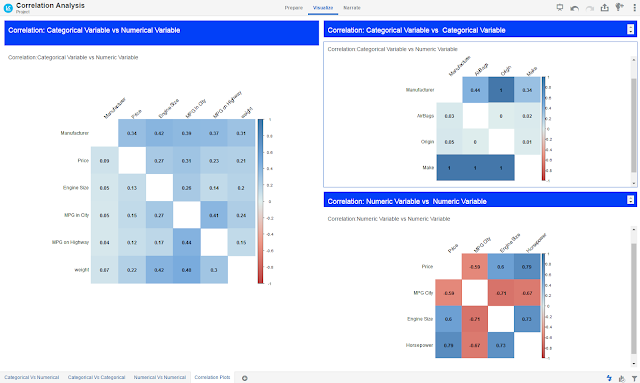Friday, February 24, 2017

OracleDV: Calculate correlation between numerical and categorical variables.

In this blog we will talk about two custom R-scripts that calculates and plots(resp) Correlation not just between two numerical variables, but between numerical and or categorical variables. Before we jump into the details about this script, let us understand what is correlation. Correlation refers to the extent to which two variables have a linear relationship with each other. Some of the famous and well known measures to compute correlation between variables include: Pearson's Product Moment coefficient, Rank correlation coefficients, Kendall and Spearman coefficients. But these coefficients work well only with numeric variables. To compute correlation between two categorical variables or between a numerical and categorical variable chi-squared test or ANOVA.

In these R-scripts we tried to address the need for a script which can compute correlation between not only two numeric variables but also between numeric and or categorical variables(num vs categorical and categorical vs categorical). Like we mentioned earlier there are two custom R-Scripts, first script computes just computes the correlation and returns the results in tabular format and 2nd script computes the correlation, plots these correlation coefficients using corrplot R-package and returns these R-visualizations.These scripts use Goodman Kruskal Algorithm (more information here) to compute correlation between num vs categorical variables and categorical vs categorical variables. To compute correlation between two numeric variables the script can use various methods like : pearson, kendall and spearman depending on users' preference. To demonstrate these scripts we have attached a sample .dva project which demonstrates how the R-Scripts can be invoked in OracleDV. You can download this script from Oracle BI Public Store.This is how your OracleDV should look like after you import the .dva project:Please note that you have to deploy R Viz(Base64Image) custom plugin before you import the .dva project.

How does this scripts work: This Script computes correlation between two variables and generates plots using corrplot R-package. The variables can either be both numeric or numeric and categorical or both categorical. This script uses two methods to calculate correlation coefficient depending on the type of input variables. Following are the methods:
1) If the variables are all numeric then the script uses one of Pearson,Kendall and Spearman methods depending on users preference.
2) For computing correlation between categorical and numerical or between categorical and categorical variables, script uses Goodman Kruskal Algorithm.
Script scans the datatype of input data frame and if all the columns are numeric then it chooses method-1 else it chooses method-2. Script returns correlation coefficient for each pair wise combination of the input columns.

Inputs:
1) id: ID to uniquely identify each column and to avoid auto aggregation.
2) column1 ... column12: Columns list for which correlation needs to be computed between each possible pair. If user needs to compute correlation between more columns, more inputs can be added to this script in exactly the same format as existing input columns.

Optional Inputs:
1) column_names: Names of the columns sent as input to the R-Script, excluding ID column. This is needed to name the columns appropriately in the output returned by R.
2) corr_method: This is applicable only if all the columns are numeric. If all the columns are numeric/metric then the script lets user choose anyone correlation method from Pearson,Kendall and Spearman.
3) plot_width: Width of the plot generated by the R-Script. Default is 400
4) plot_height: height of the plot generated by the R-Script. Default is 400

Output:
1) corr_col1: Name of first column in the pair of columns for which we are trying to compute correlation.
2) corr_col2: Name of second column in the pair of columns for which we are trying to compute correlation.
3) img* columns return the R plots in base 64 encoded image format. R Viz(base64image) custom Viz plugin parses these base64 encoded image strings and displays the image on DV Desktop canvas.

Package Dependency: corrplot, reshape, data.table, classInt, base64enc

This package contains two R-scripts:
1) R.Correlation.xml: This R-Script computes correlation between the variables.
2) R.CorrelationPlot.xml: This R-Script, in addition to computing the correlation coefficient also displays the correlation plot and converts the images to base64 encoded string formats and sends it to DV. Base64Image custom visualization converts these strings back to image.

Steps to deploy this plugin in your local Oracle DV:

1) Install Advanced Analytics feature in Oracle DV by clicking on the below icon. This will install Oracle R deployment. Alternatively you can install Advanced Analytics by running install_advanced_analytics.cmd present in <DV_INSTALL_DIRECTORY>

2) Install R-Packages:
Open R console(double click Rgui.exe present in <Advanced_Analytics_Install_Dir>\bin\x64),
install arules Package. Following are the R-commands to install:
Set Proxy:
\$ Sys.setenv(http_proxy="<your_proxy_host>:<port_number>")
set proxy appropriate to your network config.
Install Package(updated instructions):
\$ install.packages("corrplot")
\$ install.packages("reshape")
\$ install.packages("data.table")
\$ install.packages("classInt")
\$ install.packages("base64enc")
4) Copy R.Correlation.xml and R.CorrelationPlot.xml to <DV_INSTALL_DIRECTORY>\OracleBI1\bifoundation\advanced_analytics\script_repository
5) Deploy R Viz(Base64Image) Custom visualization.
6) Import the .dva project to Oracle DV. Password for the .dva is Admin123

Maz said...

Is it possible to install these R Packages on OAC instead of DV Desktop?

ORACLE ANALYTICS said...

Yes it is possible to install these packages in OAC. You have to find a writable directory and install the packages to that directory using install.packages('package_name', lib="").

Though it is possible, these packages wont be persisted when upgrade happens, That is to say it is not supported by Oracle.

Anand said...

I cannot locate the Correlation_Analysis_V1.zip on the BI Public Store. Has that been taken away? Can anyone send me the file please?

amarnath said...

I believe it is being moved and even I don't find it anywhere.